# Setup for 3 Player a Game of Food Chain Magnate

Originally generated on 12/3/2018 6:40:25 AM
A direct link to this setup is https://www.BoardgameHelpers.com/FoodChainMagnate/LoadGame.aspx?id=\$2a\$04\$8NSl5RfsbpEWsDLNlmj8Eu

## Player Setup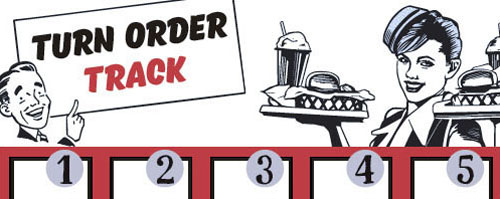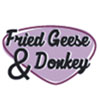## Other Setup

Bank starts with \$150.00
Remove these Billboards: #15, #16
Number of 1x Employee cards used = 1

## Map (Balanced)

Click here to view Map Tile Lettering Key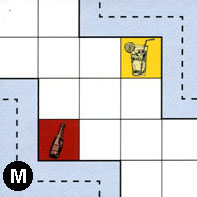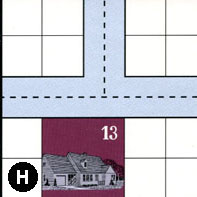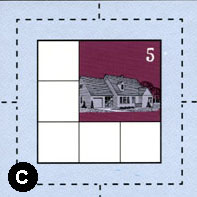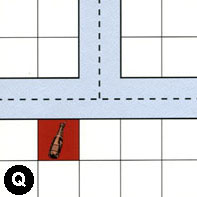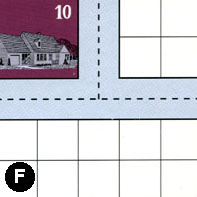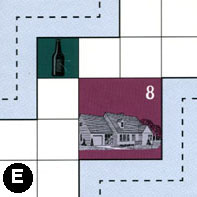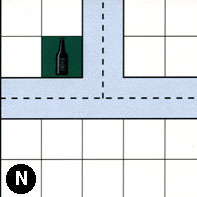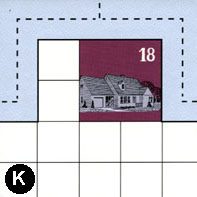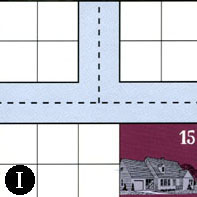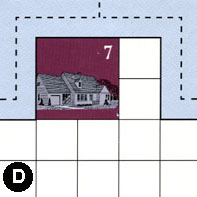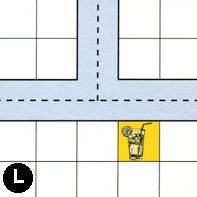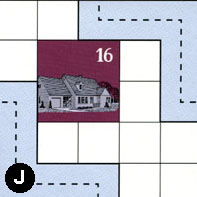### Map Stats

Map Option = Balanced
Balance Issues = Not enough path systems. Not enough drink spots. Not enough large neighborhoods. Not enough empty space in neighborhoods.
Total Number of Tiles = 12
Total Number of Starting Houses = 8
Total Number of Beer Spots = 2
Total Number of Soda Spots = 2
Total Number of Lemonade Spots = 2
Total Number of Drink Spots = 6
Number of independent path systems = 4
Number of independent neighborhoods = 18

Path System 1
Size = Medium
Number of Tiles = 7
Number of Paths = 20
Contains Loop(s) = No
Number of Starting Houses = 5
Starting Houses Pct of Map (%) = 62
Number of Beer Spots = 1
Number of Soda Spots = 1
Number of Lemonade Spots = 1
Number of Drink Spots = 3
Drink Spots Pct of Map (%) = 50
Tile Ids = C, H, J, K, M, N, Q
Paths = MEN, HWN, HNE, CWN, NWN, NNE, NWE, CWE, CNE, HWE, CNS, QWE, QWN, KWN, KNE, JWS, KWE, QNE, CWS, CES What do these mean?
Starting House Ids = 0, 5, 13, 16, 18
Path System 2
Size = Medium
Number of Tiles = 6
Number of Paths = 14
Contains Loop(s) = No
Number of Starting Houses = 5
Starting Houses Pct of Map (%) = 62
Number of Beer Spots = 0
Number of Soda Spots = 1
Number of Lemonade Spots = 1
Number of Drink Spots = 2
Drink Spots Pct of Map (%) = 33
Tile Ids = D, F, I, J, L, M
Paths = MWS, FWE, IWN, DWE, LWE, JEN, LWN, LNE, DWN, DNE, INE, IWE, FWN, FNE What do these mean?
Starting House Ids = 0, 7, 10, 15, 16
Path System 3
Size = Tiny
Number of Tiles = 1
Number of Paths = 1
Contains Loop(s) = No
Number of Starting Houses = 1
Starting Houses Pct of Map (%) = 12
Number of Beer Spots = 1
Number of Soda Spots = 0
Number of Lemonade Spots = 0
Number of Drink Spots = 1
Drink Spots Pct of Map (%) = 17
Tile Ids = E
Paths = EWN What do these mean?
Starting House Ids = 0
Path System 4
Size = Tiny
Number of Tiles = 1
Number of Paths = 1
Contains Loop(s) = No
Number of Starting Houses = 1
Starting Houses Pct of Map (%) = 12
Number of Beer Spots = 0
Number of Soda Spots = 0
Number of Lemonade Spots = 0
Number of Drink Spots = 0
Drink Spots Pct of Map (%) = 0
Tile Ids = E
Paths = EES What do these mean?
Starting House Ids = 8

Neighborhood 1
Total Size = Tiny
Number of Total Spaces = 4
Number of Empty Spaces (for new houses & gardens) = 4
Number of Beer Spots = 0
Number of Soda Spots = 0
Number of Lemonade Spots = 0
Number of Drink Spots = 0
Number of Starting Houses = 0
Starting Houses Pct of Map (%) = 0
Tile Ids = S
Neighborhood 2
Total Size = Small
Number of Total Spaces = 8
Number of Empty Spaces (for new houses & gardens) = 7
Number of Beer Spots = 1
Number of Soda Spots = 0
Number of Lemonade Spots = 0
Number of Drink Spots = 1
Number of Starting Houses = 0
Starting Houses Pct of Map (%) = 0
Tile Ids = G, S
Neighborhood 3
Total Size = Tiny
Number of Total Spaces = 4
Number of Empty Spaces (for new houses & gardens) = 4
Number of Beer Spots = 0
Number of Soda Spots = 0
Number of Lemonade Spots = 0
Number of Drink Spots = 0
Number of Starting Houses = 0
Starting Houses Pct of Map (%) = 0
Tile Ids = G
Neighborhood 4
Total Size = Tiny
Number of Total Spaces = 4
Number of Empty Spaces (for new houses & gardens) = 3
Number of Beer Spots = 0
Number of Soda Spots = 1
Number of Lemonade Spots = 0
Number of Drink Spots = 1
Number of Starting Houses = 0
Starting Houses Pct of Map (%) = 0
Tile Ids = R
Neighborhood 5
Total Size = Tiny
Number of Total Spaces = 4
Number of Empty Spaces (for new houses & gardens) = 4
Number of Beer Spots = 0
Number of Soda Spots = 0
Number of Lemonade Spots = 0
Number of Drink Spots = 0
Number of Starting Houses = 0
Starting Houses Pct of Map (%) = 0
Tile Ids = R
Neighborhood 6
Total Size = Medium
Number of Total Spaces = 9
Number of Empty Spaces (for new houses & gardens) = 5
Number of Beer Spots = 0
Number of Soda Spots = 0
Number of Lemonade Spots = 0
Number of Drink Spots = 0
Number of Starting Houses = 1
Starting Houses Pct of Map (%) = 12
Tile Ids = C
Starting House Ids = 5
Neighborhood 7
Total Size = Medium
Number of Total Spaces = 26
Number of Empty Spaces (for new houses & gardens) = 25
Number of Beer Spots = 0
Number of Soda Spots = 1
Number of Lemonade Spots = 0
Number of Drink Spots = 1
Number of Starting Houses = 0
Starting Houses Pct of Map (%) = 0
Tile Ids = F, G, O, S
Neighborhood 8
Total Size = Medium
Number of Total Spaces = 12
Number of Empty Spaces (for new houses & gardens) = 7
Number of Beer Spots = 0
Number of Soda Spots = 0
Number of Lemonade Spots = 1
Number of Drink Spots = 1
Number of Starting Houses = 1
Starting Houses Pct of Map (%) = 12
Tile Ids = G, O, P
Starting House Ids = 12
Neighborhood 9
Total Size = Tiny
Number of Total Spaces = 4
Number of Empty Spaces (for new houses & gardens) = 4
Number of Beer Spots = 0
Number of Soda Spots = 0
Number of Lemonade Spots = 0
Number of Drink Spots = 0
Number of Starting Houses = 0
Starting Houses Pct of Map (%) = 0
Tile Ids = R
Neighborhood 10
Total Size = Tiny
Number of Total Spaces = 4
Number of Empty Spaces (for new houses & gardens) = 4
Number of Beer Spots = 0
Number of Soda Spots = 0
Number of Lemonade Spots = 0
Number of Drink Spots = 0
Number of Starting Houses = 0
Starting Houses Pct of Map (%) = 0
Tile Ids = R
Neighborhood 11
Total Size = Small
Number of Total Spaces = 8
Number of Empty Spaces (for new houses & gardens) = 8
Number of Beer Spots = 0
Number of Soda Spots = 0
Number of Lemonade Spots = 0
Number of Drink Spots = 0
Number of Starting Houses = 0
Starting Houses Pct of Map (%) = 0
Tile Ids = P, R
Neighborhood 12
Total Size = Medium
Number of Total Spaces = 32
Number of Empty Spaces (for new houses & gardens) = 27
Number of Beer Spots = 1
Number of Soda Spots = 0
Number of Lemonade Spots = 0
Number of Drink Spots = 1
Number of Starting Houses = 1
Starting Houses Pct of Map (%) = 12
Tile Ids = A, K, P, T
Starting House Ids = 18
Neighborhood 13
Total Size = Medium
Number of Total Spaces = 14
Number of Empty Spaces (for new houses & gardens) = 10
Number of Beer Spots = 0
Number of Soda Spots = 0
Number of Lemonade Spots = 0
Number of Drink Spots = 0
Number of Starting Houses = 1
Starting Houses Pct of Map (%) = 12
Tile Ids = F, I
Starting House Ids = 15
Neighborhood 14
Total Size = Large
Number of Total Spaces = 40
Number of Empty Spaces (for new houses & gardens) = 31
Number of Beer Spots = 1
Number of Soda Spots = 0
Number of Lemonade Spots = 0
Number of Drink Spots = 1
Number of Starting Houses = 2
Starting Houses Pct of Map (%) = 25
Tile Ids = D, F, I, O, P, T
Starting House Ids = 7, 10
Neighborhood 15
Total Size = Tiny
Number of Total Spaces = 4
Number of Empty Spaces (for new houses & gardens) = 4
Number of Beer Spots = 0
Number of Soda Spots = 0
Number of Lemonade Spots = 0
Number of Drink Spots = 0
Number of Starting Houses = 0
Starting Houses Pct of Map (%) = 0
Tile Ids = I
Neighborhood 16
Total Size = Tiny
Number of Total Spaces = 4
Number of Empty Spaces (for new houses & gardens) = 3
Number of Beer Spots = 0
Number of Soda Spots = 0
Number of Lemonade Spots = 1
Number of Drink Spots = 1
Number of Starting Houses = 0
Starting Houses Pct of Map (%) = 0
Tile Ids = T
Neighborhood 17
Total Size = Small
Number of Total Spaces = 8
Number of Empty Spaces (for new houses & gardens) = 4
Number of Beer Spots = 0
Number of Soda Spots = 0
Number of Lemonade Spots = 0
Number of Drink Spots = 0
Number of Starting Houses = 1
Starting Houses Pct of Map (%) = 12
Tile Ids = A, T
Starting House Ids = 2
Neighborhood 18
Total Size = Tiny
Number of Total Spaces = 4
Number of Empty Spaces (for new houses & gardens) = 4
Number of Beer Spots = 0
Number of Soda Spots = 0
Number of Lemonade Spots = 0
Number of Drink Spots = 0
Number of Starting Houses = 0
Starting Houses Pct of Map (%) = 0
Tile Ids = A

v2.1.5